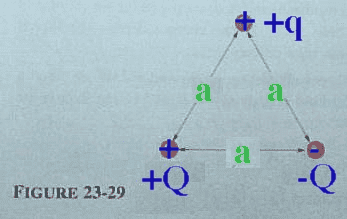# Three Point Charges, Find x-component

rlc

## Homework Statement

In the figure, three point charges are arranged in a equilateral triangle of side a= 1.40×10-2 m. Sketch the field lines due to + Q and -Q, where Q = 2.50×10-5 C , and from them determine the direction of the force that acts on +q = 5.00×10-8 C because of the presence of the other two charges. What is the x-component of the force? What is the y-component of the force?## Homework Equations

F1=K*((Q*q)/a^2)
F(x)=2|F1|*Cos(60)

## The Attempt at a Solution

I know that the y-component is 0 N.
Someone posted this problem in yahoo answers, and I tried to follow it. Something went wrong along the way, but here is the work from that solution:

*+Q=1, -Q=2, +q=3*

F1,3=(8.99E9)(5.00E-8)(2.50E-5)/(1.40E-2)=0.803 N
F2,3=(8.99E9)(5.00E-8)(-2.50E-5)/(1.40E-2)=-0.803 N

F1,3x=0.803(cos(30 degrees))=0.6951 (right)
F1,3y=0.803(cos(60))=0.4013 (down)

F2,3x=-0.803(cos(30))=-0.6951 (left)
F2,3Y=-0.803(cos(60))=-0.4013 (down

Here, I have the problem where if you combine x and y values, the answer is 0 for both of them.
Where did I go wrong, or is all of this wrong?

Mentor
F2,3=(8.99E9)(5.00E-8)(-2.50E-5)/(1.40E-2)=-0.803 N
Get rid of that negative sign. Use Coulomb's law to find the magnitude of the force, then use the signs to find the direction. Draw yourself a diagram of the forces on q.

rlc
Sorry for not replying in a while!
In the work I did, I completely forgot to square a, making all my numbers wrong.

((9E9)(q)(+Q))/(a^2)
((9E9)(5.00E-8)(2.50E-5))/(1.40E-2)^2=57.33 N (this is the x-component)

Thank you!